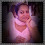## Friday 6 September 2013

### 7 Mathematical Specialities !

We are on the 6th day of The Write Tribe Festival of Words with theme '7'.
I am taking part as an external arm, and I have enjoyed writing so far.
I have also been able to read some very nice articles with 7 as the theme.

For the 6th day, I thought, why not write about 7 specialities in Mathematics ?

Ok ok...I know this is touchy ;-) Some of us didn't like Maths at school . We were scared of the teachers and had nightmares about scoring less marks or failing in the subject. We dreaded the homework and couldn't make sense of why we were being taught the area of a circle and perimeter of a square or the congruence of triangles and all that.
But this is different from all that , isn't it ? This is just a matter of infotainment, if I can use that word. Just some fun numbers :-)

Mathematics was one of my favourite subjects at school, and I was fortunate to score well in the exams. But I lost touch with the subject after my twelfth standard, when I headed for a graduation in Commerce. Since then, my connection with Mathematics has mainly been through puzzles or snippets of knowledge like this.

So, here we go :-)

The Ramanujan Number , 1729 : I am sure most of you have heard the story of Professor Hardy visiting Srinivasa Ramanujan, India's most revered mathematician, in a car numbered 1729. Ramanujan corrected Hardy's ill feeling about the number, stating that it is a unique and special number, being " the smallest number that can be expressed as the sum of 2 positive cubes in 2 ways " :-)
1^3 + 12^3 = 9^3 + 10^3 = 1729 !!
This is one of my most favourite anecdotes about the subject ! :-)

The Golden ratio, 1:1.61803 : Also called Phi, named after Phidias, the Greek sculptor, this is the natural ratio as it is found in nature. This is supposed to be the most pleasing rectangle proportion. It is found in flowers, eggs, sea shells and why, even the human body ! Any work of art created with this ratio incorporated in the measurements shall be the best! Anyone who has read Dan Brown's ' The Da Vinci Code' will be familiar with this.

12345679 : Looks weird, right ? This number, when multiplied by any 2 digit multiple of 9, will produce an answer which shall consist of only that number which multiplies with 9 to get the above multiple.
12345679 * 36 = 444444444 ( which is 36 / 9 ).

Googol : I love this number. It is 1 followed by a 100 zeroes. Even though this number has no particular mathematical significance, googol became a hit with google ! Yes, Google is a misnomer for Googol !! :-)

The Kaprekar's constant, 6174 : Named after the Indian mathematician D. R. Kaprekar. Try this trick:
- Take any four-digit number, with at least two different digits.
- Arrange the digits in ascending and then in descending order to get two four-digit numbers, adding leading zeros if necessary.
- Subtract the smaller number from the bigger number.
- Go back to step 2.
After a maximum of 7 iterations, you will be left with 6174, the Kaprekar Constant !

701 : I once came across a wonderful property of this number. This is nothing much, still it interested me.
701 = 1^0 + 2^1+3^2+4^3+5^4 :-)

5, the only odd Untouchable Number :  An untouchable number is a positive integer, that cannot be expressed as the sum of all the proper divisors of any positive integer, including the number in question :-) ; this means, the proper divisors of no positive integer adds up to 5 ;-)
Say, for example , 9. It can be expressed as the sum of divisors of 8 ( 1+2+4). But 5 is the only odd number for which this property doesn't work :-)

So happy to find out 7 peculiarities in numbers. I tried finding many more and there are many many more puzzles out there for enthusiastic mathemaniacs ! May be, some posts in future :-)

1.2.